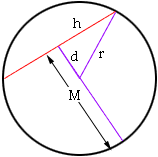From: Al Paas The level of the question would,I assume, be at the secondary level. I am no longer a student (other) ? How to determine the length of a chord given the diameter of the circle and the maximum distance from the chord to The circle? Thank you very much. Hi Al, If the radius is r, half the chord is h, and d is its distance from the center, then the Pythagorean theorem gives h2 = r2 - d2. Just replace r by (the given diam)/2, and d by M - r where M is the maximum distance from the chord to the circle. Calculate h2 = r2 - d2 take the square root of h2, and multiply by 2 to get the chord's length.Cheers, Chris Go to Math Central